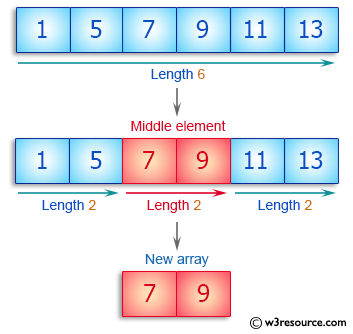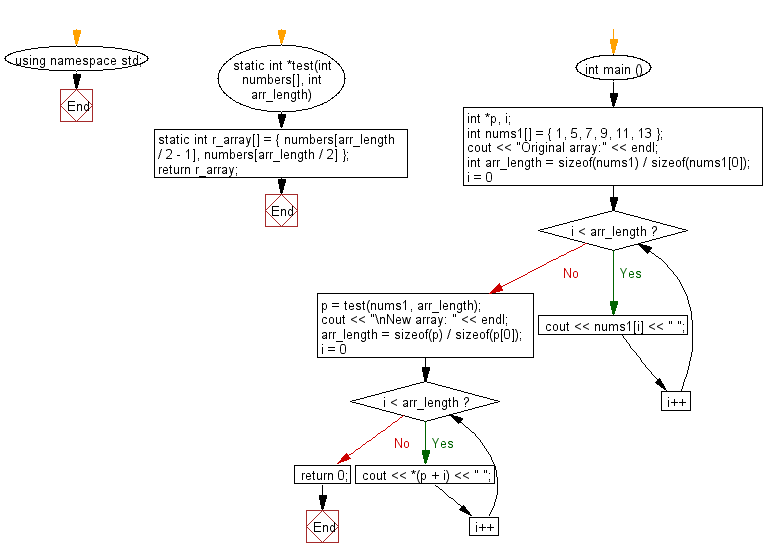﻿ C++ : New array taking two middle elements from an array# C++ Exercises: Create an array taking two middle elements from a given array of integers of length even

## C++ Basic Algorithm: Exercise-94 with Solution

Write a C++ program to create an array taking two middle elements from a given array of integers of length even.

Sample Solution:

C++ Code :

``````#include <iostream>

using namespace std;

static int *test(int numbers[], int arr_length)
{
static int  r_array[] = { numbers[arr_length / 2 - 1], numbers[arr_length / 2] };
return r_array;
}

int main () {
int *p, i;
int nums1[] = { 1, 5, 7, 9, 11, 13 };
cout << "Original array:" << endl;
int arr_length = sizeof(nums1) / sizeof(nums1);
for ( i = 0; i < arr_length; i++ ) {
cout << nums1[i] << " ";
}
p =  test(nums1, arr_length);
cout << "\nNew array: " << endl;
arr_length = sizeof(p) / sizeof(p);
for ( i = 0; i < arr_length; i++ ) {
cout << *(p + i) << " ";
}
return 0;
}
``````

Sample Output:

```Original array:
1 5 7 9 11 13
New array:
7 9
```

Pictorial Presentation:Flowchart:C++ Code Editor: